# DAV Class 5 Maths Chapter 4 Worksheet 6 Solutions

The DAV Class 5 Maths Book Solutions Pdf and DAV Class 5 Maths Chapter 4 Worksheet 6 Solutions of Fractional Numbers offer comprehensive answers to textbook questions.

## DAV Class 5 Maths Ch 4 Worksheet 6 Solutions

Question 1.
Multiply.
(a) $$\frac{1}{3}$$ × 2
Solution:
$$\frac{1}{3}$$ × 2
= $$\frac{1 \times 2}{3}$$
= $$\frac{2}{3}$$
(Product of whole number and numerator of a fractional number)

(b) $$\frac{5}{8}$$ × 9
Solution:
$$\frac{5}{8}$$ × 9
= $$\frac{5 \times 9}{8}$$
= $$\frac{45}{8}$$
= 5$$\frac{5}{8}$$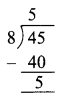(Product of whole number and numerator of a fractional number)(c) 4$$\frac{1}{2}$$ × 4
Solution:
4$$\frac{1}{2}$$ × 4
= $$\frac{9}{2}$$ × 4
= $$\frac{9 \times 4}{2}$$
= $$\frac{36}{2}$$
= 18

(d) 9$$\frac{1}{3}$$ × 27
Solution:(Product of whole number and numerator of a fractional number)

(e) 10$$\frac{1}{10}$$ × 15
Solution: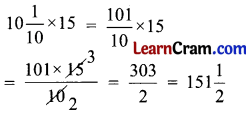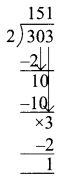(Product of whole number and numerator of a fractional number)

(f) 6 × $$\frac{4}{15}$$
Solution: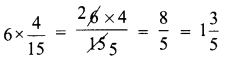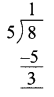(Product of whole number and numerator of a fractional number)(g) 100 × 3$$\frac{1}{10}$$
Solution: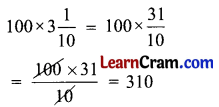(Product of whole number and numerator of fractional number)

(h) 52 × 2$$\frac{1}{13}$$
Solution:(Product of whole number and numerator of fractional number)

(i) 49 × 7$$\frac{1}{7}$$
Solution: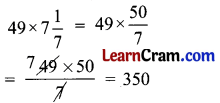(Product of whole number and numerator of fractional number)

(j) 3$$\frac{5}{8}$$ × 32
Solution: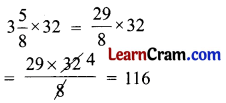(Product of whole number and numerator of fractional number)(k) 45 × 2$$\frac{1}{9}$$
Solution:(Product of whole number and numerator of fractional number)

(l) 50 × $$\frac{17}{15}$$
Solution: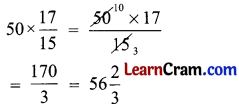(Product of whole number and numerator of fractional number)

DAV Class 5 Maths Chapter 4 Worksheet 6 Notes

Example 1.
Multiply 4 and $$\frac{1}{5}$$
Solution:
It means we have to find what is 4 times $$\frac{1}{5}$$
We know multiplication is repeated addition.
Therefore $$4 \times \frac{1}{5}=\frac{1}{5}+\frac{1}{5}+\frac{1}{5}+\frac{1}{5}=\frac{4}{5}$$
Let us take rectangular strip of paper divided into 5 equal parts.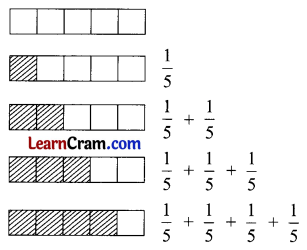Therefore, 4 × $$\frac{1}{5}$$ or we can use the quick method
4 × $$\frac{1}{5}$$ = $$\frac{4 \times 1}{5}$$ = $$\frac{4}{5}$$Example 2.
Multiply $$\frac{1}{2}$$ and 3.
Solution:
Let us consider a rectangle divided into 3 equal parts.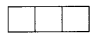We further divide these 3 parts into 6 equal parts.$$\frac{1}{2}$$ of 6 parts will be 3 equal parts (shaded portion)
Thus $$\frac{1}{2}$$ of 3 will be $$\frac{3}{2}$$ (three out of 2 equal parts)
Thus $$\frac{1}{2}$$ of 3 = $$\frac{3}{2}$$
or $$\frac{1}{2}$$ × 3 = $$\frac{1 \times 3}{2}$$ = $$\frac{3}{2}$$
Combining the two results we get
3 × $$\frac{1}{2}$$ = $$\frac{1}{2}$$ × 3 = $$\frac{3}{2}$$

Example 3.
Multiply $$\frac{2}{7}$$ and 3.
Solution:
3 × $$\frac{2}{7}$$ = $$\frac{2 \times 3}{7}$$ = $$\frac{6}{7}$$
(Product of whole number and numerator of a fractional number)

Remember: In order to get the product of whole number and a fractional number, we multiply the whole number and numerator of the fractional number. Denominator remains the same.